Chemguide: Support for CIE A level Chemistry ``` ``` Learning outcome 24: Electrochemistry 24.2: Standard electrode potentials ``` ``` Learning outcomes 24.2.8 and 24.2.9 These statements deal with the effect of concentration on electrode potentials. ``` ``` Statement 24.2.8 This statement shows why you would expect electrode potentials to vary with concentration. Let's look at this with a couple of simple examples. We have discussed this copper half-equation before in this section: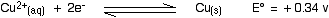For this E° value to apply, the copper(II) ions would have to have a concentration of 1 mol dm-3. What if the copper(II) ion solution was much more dilute? This is an equilibrium we are talking about. If you decrease the concentration of something in an equilibrium, the position of equilibrium shifts in such a way as to counteract the change (Le Chatelier's Principle). In this case, then, the position of equilibrium moves to the left, tending to increase the concentration of the copper ions again. But the E° value gives a measure of the position of the equilibrium. If the position of the equilibrium moves to the left, then the electrode potential becomes less positive. (It is no longer a standard electrode potential because the concentrations are no longer standard.) Suppose you have a half-equation involving two ions, such as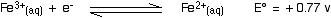If you decrease the concentration of the two ions by the same amount, then it will make no difference to the electrode potential. Any tendencies for the equilibrium position to move will just cancel out. ``` ``` For a more complicated case, look again at a part of the page about making predictions using electrode potentials. What you are interested in is the second of the two examples where the E° value seems to give the wrong answer, just over half-way down the page. ``` ``` Statement 24.2.9 This statement is about calculating how the electrode potential changes when you change concentrations. It makes use of a version of the Nernst equation. In the form that CIE want for their A level exam, the Nernst equation is: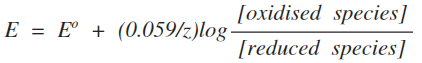``` ``` Example 1 It is easier to explain what this means with a simple example, involving the electrode potential of the Fe3+ / Fe2+ system.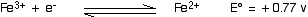This E° value applies when all the ions (both Fe3+ and Fe2+) are present at 1 mol dm-3. What happens if they aren't? Suppose the concentration of the Fe3+ ions is 1.0 mol dm-3, and the Fe2+ ions is 0.20 mol dm-3 In the Nernst equation, the terms "[oxidised species]" and "[reduced species]" are used. The oxidised species is the one in the higher oxidation state - in this case the Fe3+ ions. The reduced species is the one in the lower oxidation state - the Fe2+ ions. The square brackets show concentrations in mol dm-3. Note:  If you go on to do chemistry at a higher level, you will find that we shouldn't really be talking about concentrations here - we should be talking about "activities". These are too difficult to deal with at this level, and concentrations are used (inaccurately) instead. Strictly, you can only use concentrations for very dilute solutions. So, we have the terms in the "log" expression. The "z" refers to the number of electrons transferred in the reaction we are talking about - in this case, 1.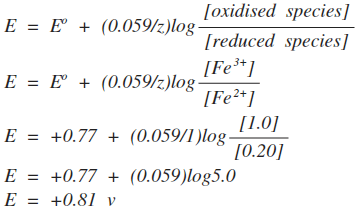Note:  If you aren't happy about logs, just work out the value in the log expression first - in this case giving the answer 5. Then press the "log" button on your calculator. Now multiply that by 0.059, and add 0.77 to that answer. If your calculator doesn't give the answer above, it obviously handles logs differently. Read the instruction book! Is this consistent with what you would expect using Le Chatelier's Principle and looking at the equilibrium? Yes! If you decrease the concentration of iron(II) ions, the equilibrium will shift to counter the change. It will move to the right. The electrode potential is a measure of the position of equilibrium. If it moves to the right, it will become more positive. If you know about logs, you will realise that as long as the concentrations of the two ions are the same, the electrode potential will be the same as the standard electrode potential. Log(1) is zero, and so the whole of that part of the equation disappears. That is also consistent with Le Chatelier's Principle. Changing both of the ion concentrations by the same amount will have no effect on the position of equilibrium. ``` ``` Example 2 This relates to the Cu2+ / Cu system.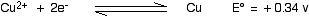This is very similar to the first example, but introduces a new problem. The oxidised species is Cu2+; the reduced species is Cu. How can you have a concentration of Cu in mol dm-3? Well, actually, you can't! But we shouldn't be talking about this in terms of concentration anyway. In terms of activities, the activity of a metal like copper is 1. The net effect of that is that the Nernst equation simplifies as you will see in this example. Note:  Don't worry about this. It is impossible to explain properly at this level. Just accept that if you have a metal, you can treat its concentration as if it was 1. What is the electrode potential of copper in a solution containing 0.10 mol dm-3 Cu2+?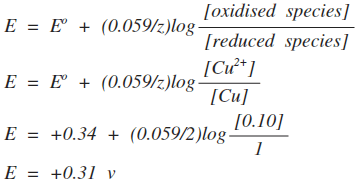Notice that this time, we have had to divide the 0.059 by 2. That's because we are transferring two electrons. You should be able to work out for yourself whether this change on diluting the copper(II) ion solution is consistent with Le Chatelier. It is! The position of the equilibrium will lie slightly further to the left. ``` ``` Example 3 This relates to the Cl2 / Cl- system.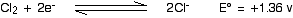The oxidised species this time is Cl2, and the reduced species is Cl-. This time you are faced with a "concentration" of chlorine. Once again, you take that as being 1, and again there is no easy way of explaining why that is. The new thing this time lies in the expresion for the chloride ions. Although the form of the Nernst equation in the CIE syllabus doesn't show it, the concentration terms have to be handled exactly as you would in an equilibrium expression. Because of the 2 in front of the chloride ions in the equation, you have to square their concentration term. So, we'll calculate the electrode potential for a non-standard solution of chloride ions containing 0.10 mol dm-3 of Cl-.Notice that we have had to divide the 0.059 by 2 again because 2 electrons are being transferred. ``` ``` Warning! From past papers, it seems that you will have to remember the Nernst equation as well as use it. Either get used to it by practising a whole lot of questions, or else make a note to learn it the night before the exam. ``` ``` Go to the Section 24 Menu . . . To return to the list of learning outcomes in Section 24 Go to the CIE Main Menu . . . To return to the list of all the CIE sections Go to Chemguide Main Menu . . . This will take you to the main part of Chemguide. © Jim Clark 2020